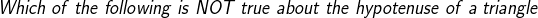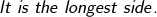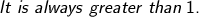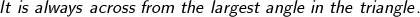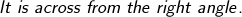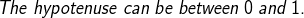# PSAT Math : How to find the length of the hypotenuse of a right triangle : Pythagorean Theorem

## Example Questions

### Example Question #971 : High School Math

If the base of a right triangle is 5 cm long and the height of the triangle is 7 cm longer than the base, what is the length of the third side of the triangle in cm?Explanation:

Find the height of the triangle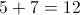Use the Pythagorean Theorem to solve for the length of the third side, or hypotenuse.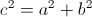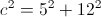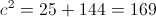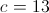### Example Question #251 : Triangles

Given the right triangle in the diagram, what is the length of the hypotenuse?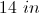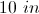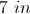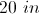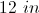Explanation:

To find the length of the hypotenuse use the Pythagorean Theorem: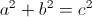Where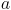andare the legs of the triangle, and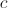is the hypotenuse.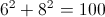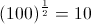The hypotenuse is 10 inches long.

### Example Question #21 : How To Find The Length Of The Hypotenuse Of A Right Triangle : Pythagorean Theorem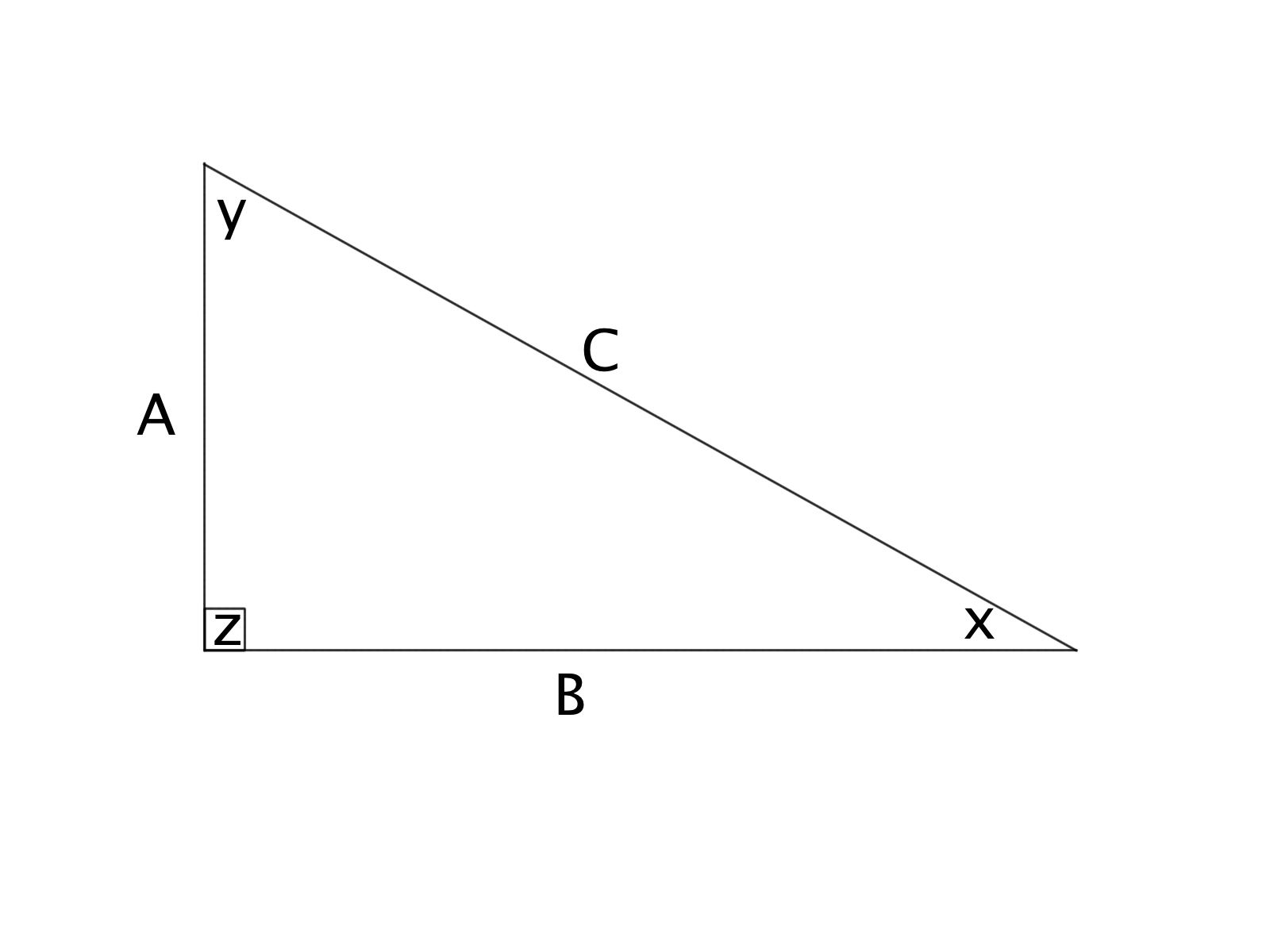Triangle ABC is a right triangle. If the length of side A = 3 inches and C = 5 inches, what is the length of side B?

1 inches

6 inches

1/2 inches

4 inches

4.5 inches

4 inches

Explanation:

Using the Pythagorean Theorem, we know that.

This gives: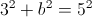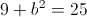Subtracting 9 from both sides of the equation gives: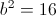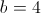inches### Example Question #21 : How To Find The Length Of The Hypotenuse Of A Right Triangle : Pythagorean TheoremTriangle ABC is a right triangle. If the length of side A = 8 inches and B = 11 inches, find the length of the hypoteneuse (to the nearest tenth).

13.7 inches

185 inches

184 inches

14.2 inches

13.6 inches

13.6 inches

Explanation:

Using the Pythagrean Theorem, we know that.

This tells us: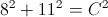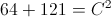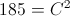Taking the square root of both sides, we find that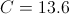inches

### Example Question #411 : GeometryGiven:

A = 6 feet

B = 9 feet

What is the length of the hypoteneuse of the triangle (to the nearest tenth)?

10.5 feet

10.2 feet

10.8 feet

10.1 feet

10.6 feet

10.8 feet

Explanation:

Using the Pythagrean Theorem, we know that.

This tells us: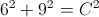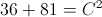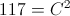Taking the square root of both sides, we find that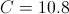### Example Question #21 : How To Find The Length Of The Hypotenuse Of A Right Triangle : Pythagorean TheoremGiven:

A = 2 miles

B = 3 miles

What is the length of the hypoteneuse of triangle ABC, to the nearest tenth?

3.6 miles

3.2 miles

3.7 miles

3.4 miles

3.5 miles

3.6 miles

Explanation:

Using the Pythagrean Theorem, we know that.

This tells us: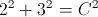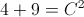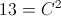Taking the square root of both sides, we find that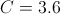### Example Question #51 : Right Triangles

Given that two sides of a right triangle measure 2 feet and 3 feet, respectively, with a hypoteneuse of x, what is the perimeter of this right triangle (to the nearest tenth)?

6.4 feet

3.6 feet

18 feet

8.6 feet

9.4 feet

8.6 feet

Explanation:

Using the Pythagrean Theorem, we know that.

This tells us:Taking the square root of both sides, we find thatTo find the perimeter, we add the side lengths together, which gives us that the perimeter is: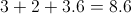### Example Question #27 : How To Find The Length Of The Hypotenuse Of A Right Triangle : Pythagorean Theorem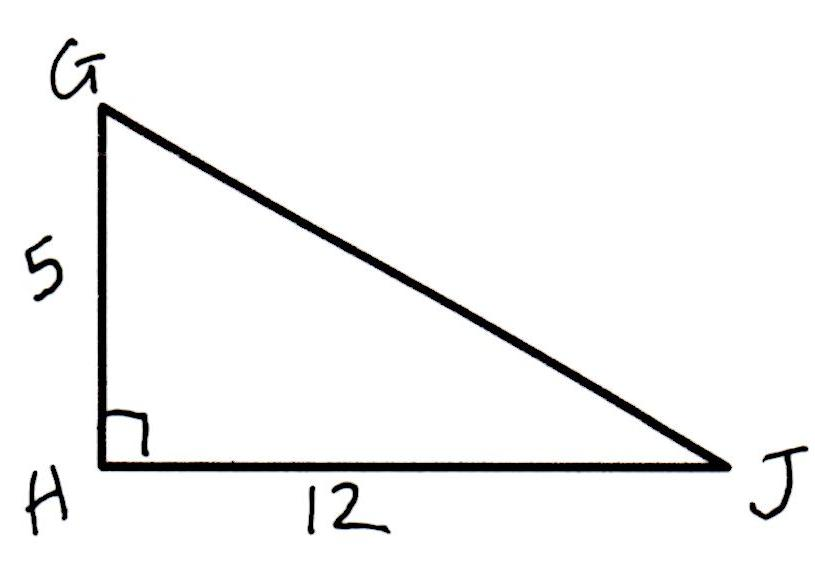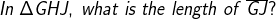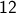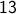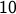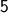Explanation: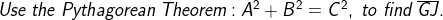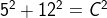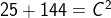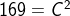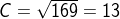### Example Question #111 : Geometry

Kathy and Jill are travelling from their home to the same destination. Kathy travels due east and then after travelling 6 miles turns and travels 8 miles due north. Jill travels directly from her home to the destination. How miles does Jill travel?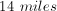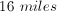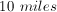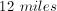Explanation:

Kathy's path traces the outline of a right triangle with legs of 6 and 8. By using the Pythagorean Theorem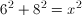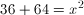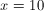miles

### Example Question #29 : How To Find The Length Of The Hypotenuse Of A Right Triangle : Pythagorean Theorem